# Chemical Reactions Chemical Reactions 10 1 Reactions and

• Slides: 23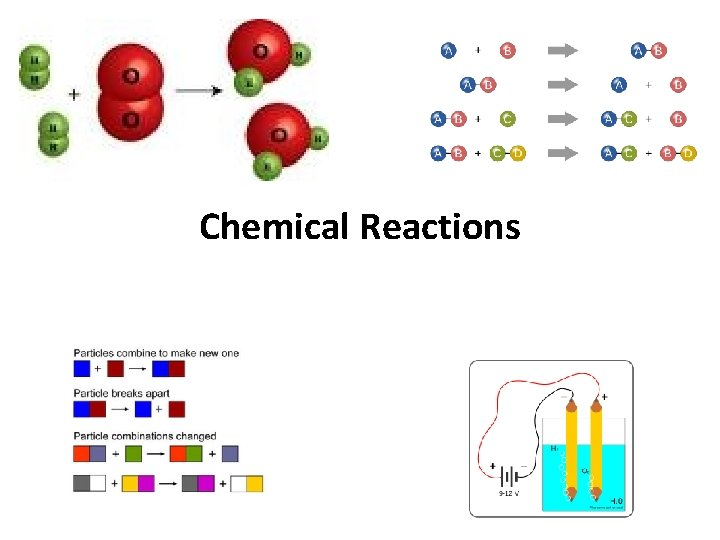Chemical Reactions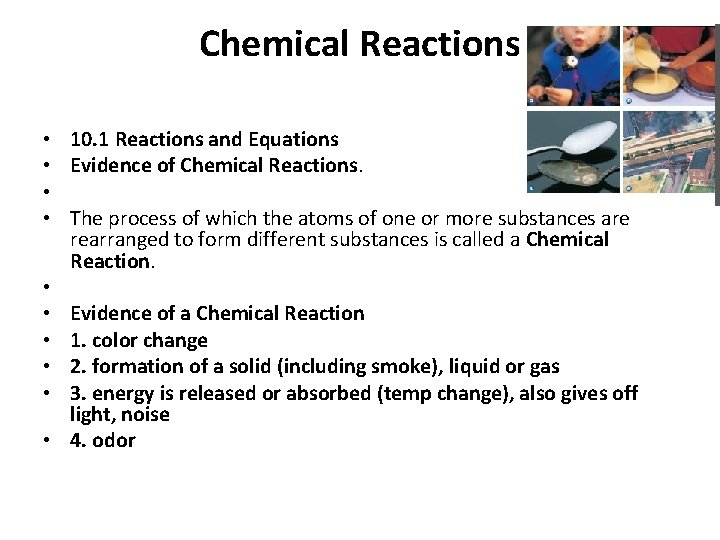Chemical Reactions • 10. 1 Reactions and Equations • Evidence of Chemical Reactions. • • The process of which the atoms of one or more substances are rearranged to form different substances is called a Chemical Reaction. • • Evidence of a Chemical Reaction • 1. color change • 2. formation of a solid (including smoke), liquid or gas • 3. energy is released or absorbed (temp change), also gives off light, noise • 4. odor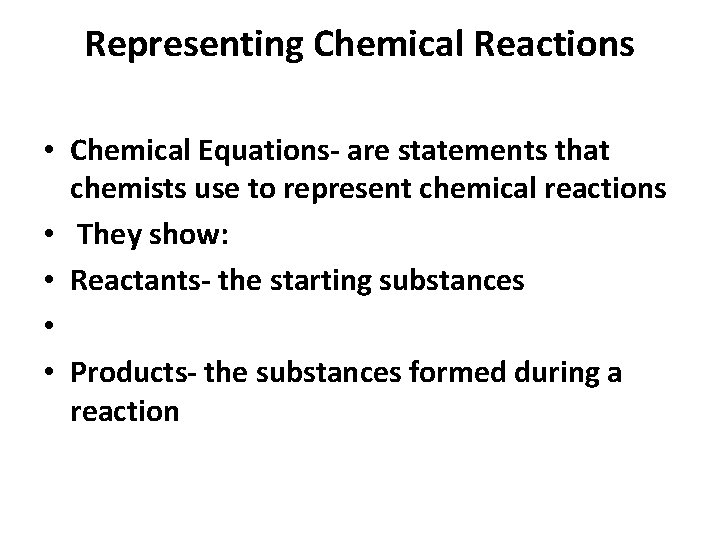Representing Chemical Reactions • Chemical Equations- are statements that chemists use to represent chemical reactions • They show: • Reactants- the starting substances • • Products- the substances formed during a reaction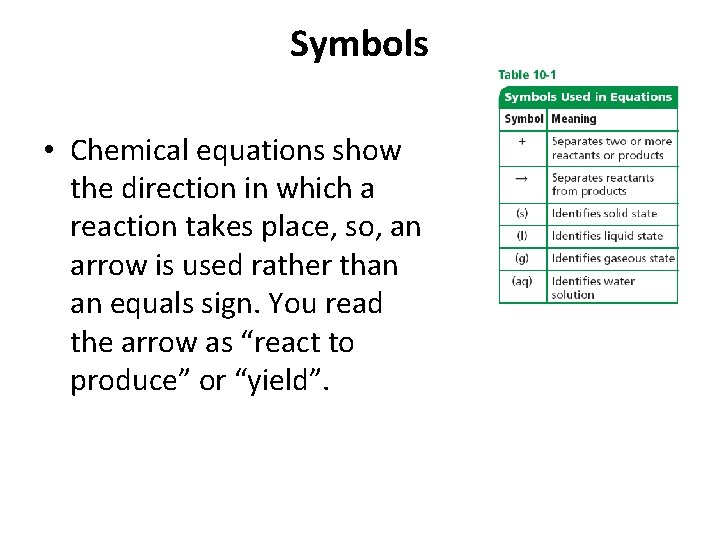Symbols • Chemical equations show the direction in which a reaction takes place, so, an arrow is used rather than an equals sign. You read the arrow as “react to produce” or “yield”.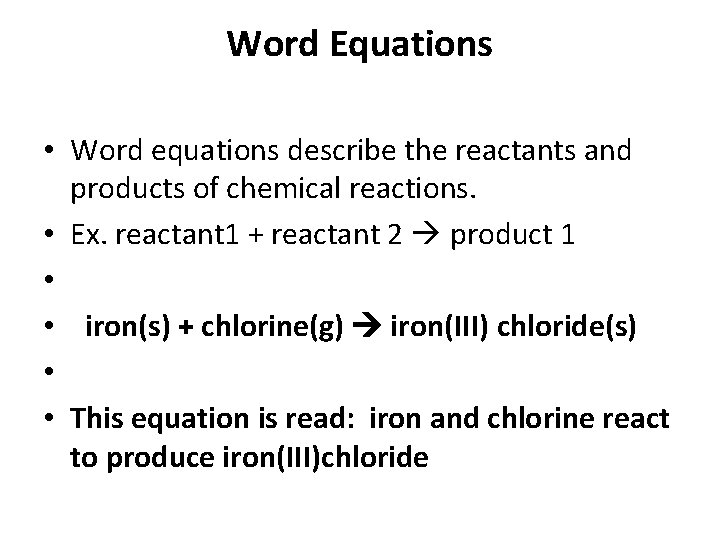Word Equations • Word equations describe the reactants and products of chemical reactions. • Ex. reactant 1 + reactant 2 product 1 • • iron(s) + chlorine(g) iron(III) chloride(s) • • This equation is read: iron and chlorine react to produce iron(III)chlorideSkeleton Equations • A skeleton equation uses chemical formulas rather than words to identify the reactants and the products. • Ex: iron(s) + chlorine(g) iron(III) chloride(s) • Fe(s) + Cl 2(g) Fe. Cl 3 (s)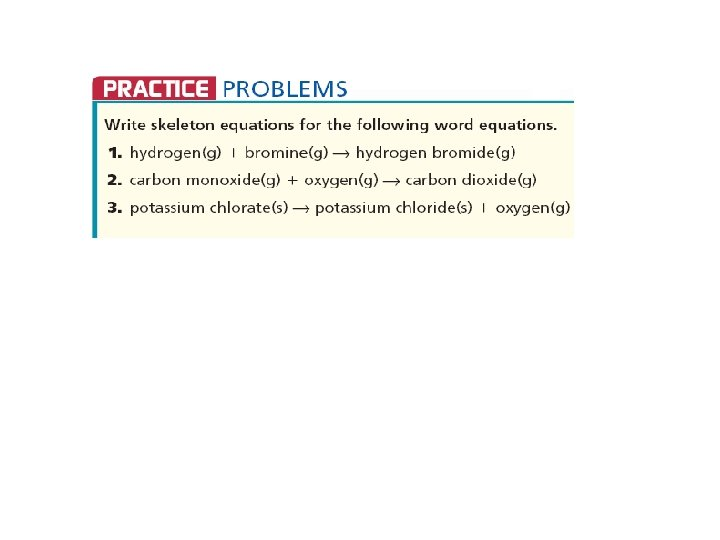Writing Ionic Compound Formulas Review. 1. Write the symbols 2. Write the charges 3. Cross the charges from top to bottom. 4. Remove the charges 5. Simplify the numbers (ratios) and remove the ones. • Reminder: treat polyatomic ions as one ion. • • •Balancing Chemical Equations. The law of conservation of matter states that, in a chemical change, matter is neither created nor destroyed.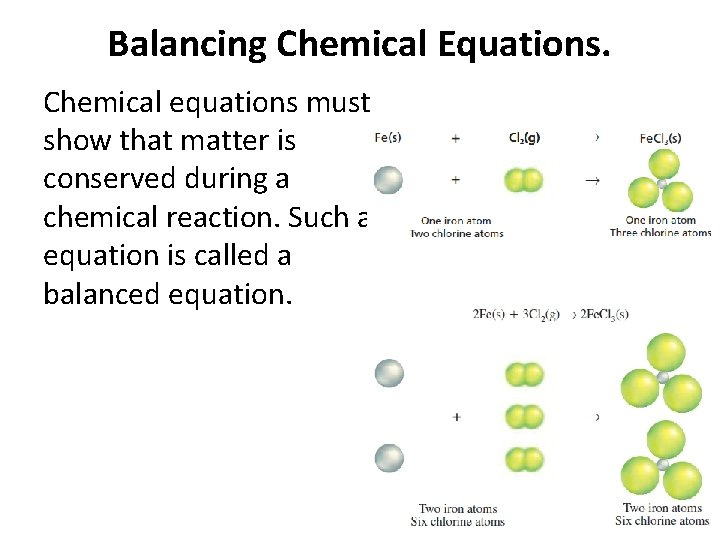Balancing Chemical Equations. Chemical equations must show that matter is conserved during a chemical reaction. Such an equation is called a balanced equation.Balancing Chemical Equations. To balance an equation you must find the correct coefficients for the chemical formulas in the skeletal equations. A coefficient in a chemical equation is the number written in front of a reactant or product.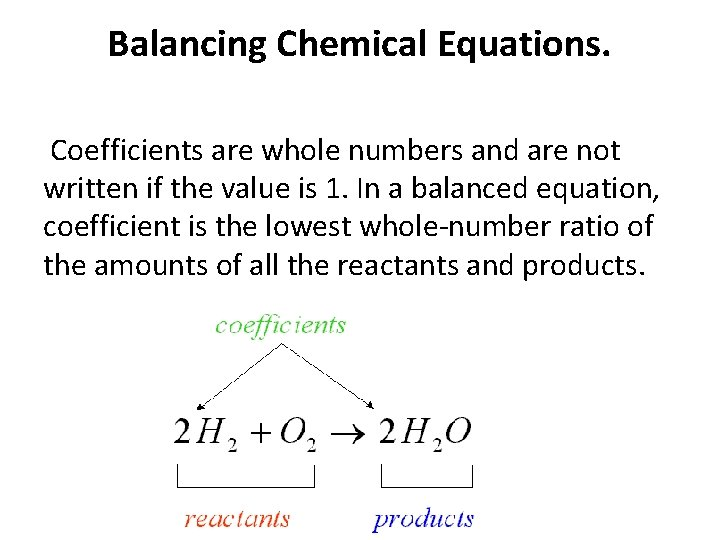Balancing Chemical Equations. Coefficients are whole numbers and are not written if the value is 1. In a balanced equation, coefficient is the lowest whole-number ratio of the amounts of all the reactants and products.Steps for Balancing Equations • 1. Write the skeletal equation for the reaction. • 2. Count the atoms of the elements in the reactants. • 3. Count the elements in the products. • 4. Change the coefficients to make the number of atoms of each element equal on both • sides of the equation. • 5. Write the coefficients in their lowest possible ratio. • 6. Check your work.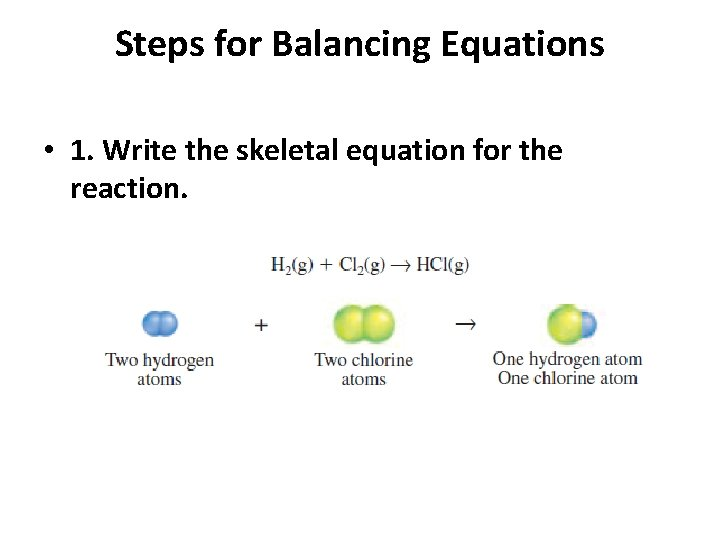Steps for Balancing Equations • 1. Write the skeletal equation for the reaction.Steps for Balancing Equations • 2. Count the atoms of the elements in the reactants.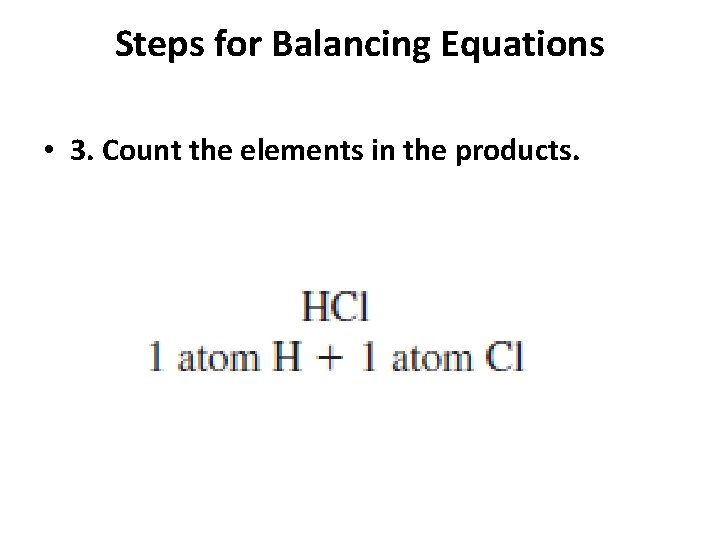Steps for Balancing Equations • 3. Count the elements in the products.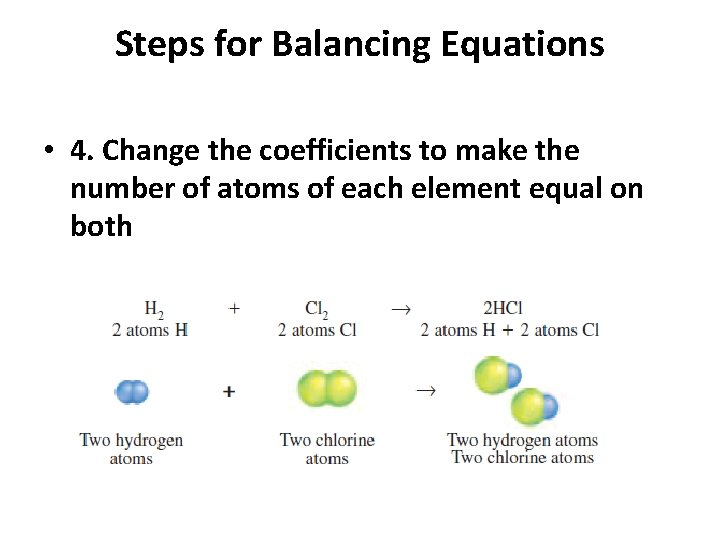Steps for Balancing Equations • 4. Change the coefficients to make the number of atoms of each element equal on both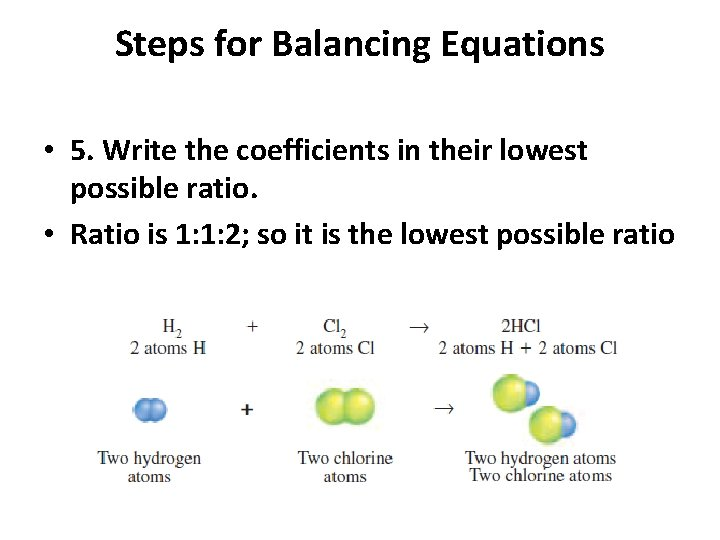Steps for Balancing Equations • 5. Write the coefficients in their lowest possible ratio. • Ratio is 1: 1: 2; so it is the lowest possible ratio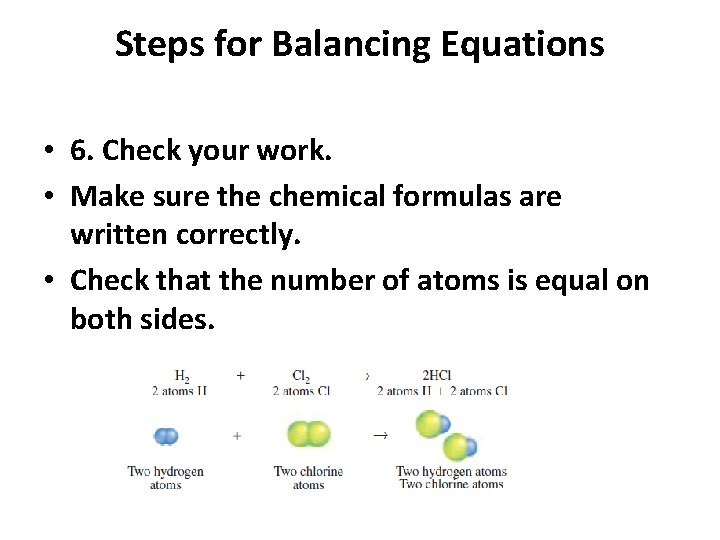Steps for Balancing Equations • 6. Check your work. • Make sure the chemical formulas are written correctly. • Check that the number of atoms is equal on both sides.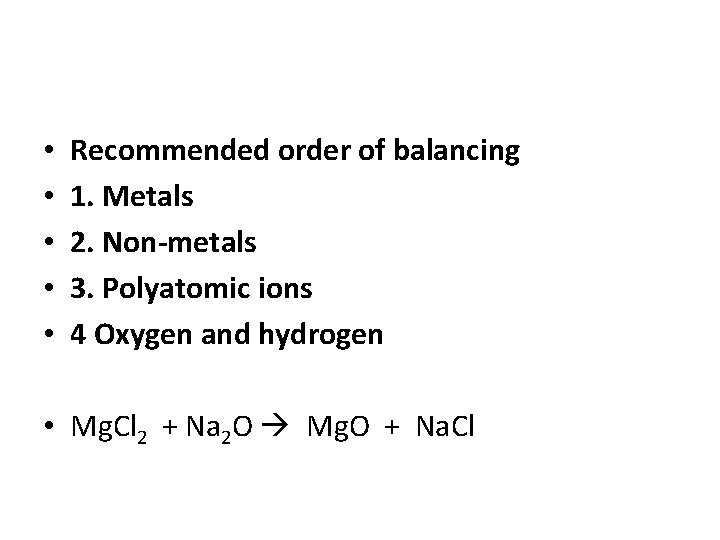• • • Recommended order of balancing 1. Metals 2. Non-metals 3. Polyatomic ions 4 Oxygen and hydrogen • Mg. Cl 2 + Na 2 O Mg. O + Na. ClTry to balance these equations!Do Now • In your packet: Write a skeletal equation of the following reactions and then balance.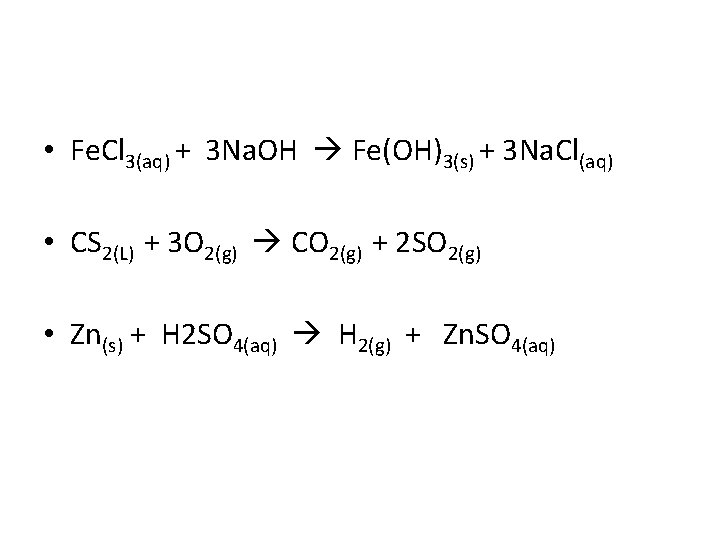• Fe. Cl 3(aq) + 3 Na. OH Fe(OH)3(s) + 3 Na. Cl(aq) • CS 2(L) + 3 O 2(g) CO 2(g) + 2 SO 2(g) • Zn(s) + H 2 SO 4(aq) H 2(g) + Zn. SO 4(aq)